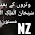### ASP.Net Reading Microsoft Project MPP File using interop.

ASP.Net Reading Microsoft Project MPP File using interop.
Its easy but works only on running at machine having visual studio installed. Below is a program made for importing data from MPP to SAP PS Module. The code can read data from MPP File and bind to a Grid for display.

Imports Microsoft.Office.Interop.MSProject

Dim appclass As New Application

Dim oMissing = Type.Missing
Dim bopen = appclass.FileOpenEx(Server.MapPath("./upload/") & "mspfile.mpp", True, PjMergeType.pjDoNotMerge, oMissing, oMissing, oMissing, oMissing, oMissing, oMissing, oMissing, oMissing, PjPoolOpen.pjDoNotOpenPool, oMissing, oMissing, oMissing, oMissing)

If bopen = False Then
lblMsg.Text = "File not opened"
Exit Sub
End If

appclass.Visible = False
Dim project As Project = appclass.ActiveProject

Dim temp As String = "
"

Dim id As Integer = 1
'########### ' Store task id in array
' 0001 3 Boiler Erection Start 18FS+183 days
i = 1

'We require 3 no of level. Get max no of level in mpp file
' Rule if maxlevel is 4 > level 3 & 4 ->3 and level 1 & 2 > 2 and 0 > 1
Dim maxlevel = 0
Dim currentlevel = 0
Dim wbs = ""
If Not task Is Nothing Then
If Not task.Summary Then  'remove yes
currentlevel = task.WBS.ToString.Split(".").Count     '1 will store 3
If maxlevel < currentlevel Then
maxlevel = currentlevel
End If
i = i + 1
End If
End If
Next
' temp = " WBS " & wbs & " MaxLevel " & maxlevel.ToString
Dim basewbs = txtWBS.Text   '"IN-C24320.2.19.04" '"IN-C24320.2.12"  ' "IN-C24320.2.19.10"
Dim baselevel = basewbs.ToString.Split(".").Count  'Total level should not exceed 7/8. expected is 6
Dim taskwbs = ""
'' to generate levels AA,AB,AC ... etc
Dim AX() = "ABCDEFGHIJKLMNOPQRSTUVWXYZ".ToCharArray
Dim XA() = "ABCDEFGHIJKLMNOPQRSTUVWXYZ".ToCharArray
Dim AXcount = 0
Dim XAcount = 0
Dim j = 0
Dim mytable = New System.Data.DataTable
Dim myrow = mytable.NewRow
If Not task Is Nothing Then
'Rule : If summary is true then unique wbs else copy wbs of the parent

Dim s() = task.WBS.ToString.Split(".")
Dim t = ""
For j = 0 To s.Length - 1
If Not j = 0 And baselevel + j <= 6 Then  'j=0 first octet not required, and j
t = t & "." & s(j).PadLeft(2, "0")
ElseIf baselevel + j > 6 Then
t = Left(t, t.Length - 3) & "." & AX(AXcount).ToString & XA(XAcount).ToString
XAcount = XAcount + 1
If XAcount = 25 Then  ' reached AZ so reset for BA
AXcount = AXcount + 1  'A to B
XAcount = 0  'Z to A
End If
End If

Next
taskwbs = basewbs & t

myrow(1) = (baselevel + j - 1).ToString
myrow(5) = (Split(taskwbs, ".").Count).ToString
id = id + 1
Else
'   temp = temp & " " & task.ID.ToString.PadLeft(4, "0") & "" & (Split(taskwbs, ".").Count).ToString & "" & (baselevel + j - 1).ToString & "" & taskwbs & "" & task.Name & "" & task.WBS & "" & task.Summary & " "
myrow(1) = (baselevel + j - 1).ToString
myrow(5) = (Split(taskwbs, ".").Count).ToString
End If
End If
Next
GridView1.DataSource = mytable
GridView1.DataBind()
'  Label1.Text = "Total Task " & project.Tasks.Count & temp & "
"
' Make sure to clean up and close the file
appclass.FileCloseAll(PjSaveType.pjDoNotSave)

EndMeredith Derek said…
Try .NET library from Aspose for reading Microsoft Project files in MPP/XML format. It also allows you to create and manipulate MS Project files in .NET.